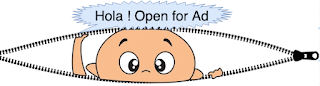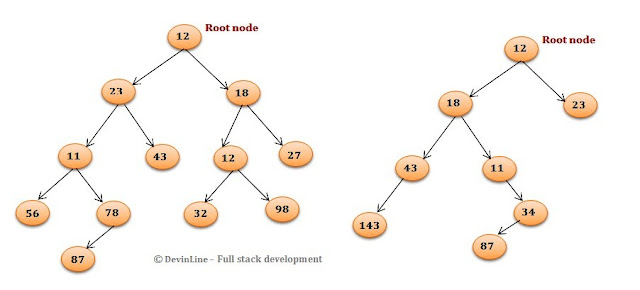## Latest Posts

Problem Statement :- Find diameter of binary tree in Java.The diameter of binary tree or width of tree - number of nodes of longest path between two leaves of tree.Left Binary tree(Tree 1) and Right binary tree(Tree 2)
Diameter of binary tree(left side) :-    8
Diameter of binary tree(right side) :-  6

Diameter of binary tree - Time complexity O(n^2)Algorithm : Idea here is to find height of left and right subtree and compare with current diameter and update diameter accordingly. Run time complexity is of order O(n^2), since height is calculated as separate method and nodes are visited more than once.
1. Check for empty tree, if true return zero.
2. Find left and right subtree height say leftHeight and rightheight.
3. Recursively call for finding diameter of left and right subtree and store in variable leftDiam and rightDim, calculate curMaxDiam as maximum of leftDiam  and rightDim.
4. Return maximum of current diameter and (leftHeight + rightheight+1) 1 added for root.
Sample code for finding diameter of binary tree - time complexity O(n^2)
```/* Recursive approach - Diameter of binary tree with time complexity O(n2) */
public int findDiameterOfBinaryTreeRecursiveWithOn2(Node root) {
if (root == null)
return 0;
/* Find left and right subtree height of given (node)root */
int leftHeight = findHeightOfTree(root.leftChild);
int rightheight = findHeightOfTree(root.rightChild);
/* Find diameter of left and right subtree */
int leftDiam = findDiameterOfBinaryTreeRecursiveWithOn2(root.leftChild);
int rightDim = findDiameterOfBinaryTreeRecursiveWithOn2(root.rightChild);
int curMaxDiam = leftDiam > rightDim ? leftDiam : rightDim;

/* compare current max with sum of L and R tree height */
return Math.max((leftHeight + rightheight + 1), curMaxDiam);
}```

Diameter of binary tree - Time complexity O(n)
Algorithm-2 :- We can optimize above algorithm(finding height of subtree as part of same method) and run time complexity turned out to be order of  O(n). Idea here is to store height and diameter with respect to each node and update height by returning height from child nodes.Update diameter by comparing left, right and diameter including root.
1. Make recursive call to find height and diameter of left and right subtree
2. Update height with respect to current node.
3. Find max of left, right diameter and diameter considering root
4. Update DiameterAndHeightArr and height.
Sample program for find diameter of binary tree - time complexity O(n)
```/* Recursive approach - Diameter of binary tree with time complexity O(n) */
public int[] findDiameterOfBinaryTreeRecursiveWithOn(Node root) {
/* initialize the height and diameter as 0,0 in DiameterAndHeightArr)
Height index- 0 and Diam Index -1  */
int DiameterAndHeightArr[] = { 0, 0 };
/*For empty tree return default array */
if(root == null)
return DiameterAndHeightArr;
else {
/* Recursive call to find height and diameter of left and right subtree */
int[] leftSubtreeResult = findDiameterOfBinaryTreeRecursiveWithOn(
root.leftChild);
int[] rightSubtreeResult = findDiameterOfBinaryTreeRecursiveWithOn(
root.rightChild);
/*Update height with respect to current node*/
int height = Math.max(leftSubtreeResult, rightSubtreeResult) + 1;
int leftDiameter = leftSubtreeResult;
int rightDiameter = rightSubtreeResult;
int rootDiameter = leftSubtreeResult + rightSubtreeResult + 1;
/*Find max of left, right diameter and diameter considering root*/
int finalDiameter = getMax(leftDiameter, rightDiameter,
rootDiameter);
// Update DiameterAndHeightArr and height
DiameterAndHeightArr = height;
DiameterAndHeightArr = finalDiameter;
return DiameterAndHeightArr;
}
}
public int getMax(int a, int b, int c) {
return Math.max(a, Math.max(b, c));
}
```

#### Complete sample program- find diameter of binary tree

```package com.devinline.trees;
public class DiameterOfBinaryTree {
static int diameter = 0;

/* Recursive approach - Diameter of binary tree with time complexity O(n^2) */
public int findDiameterOfBinaryTreeRecursiveWithOn2(Node root) {
if (root == null)
return 0;
/* Find left and right subtree height of given (node)root */
int leftHeight = findHeightOfTree(root.leftChild);
int rightheight = findHeightOfTree(root.rightChild);
/* Find diameter of left and right subtree */
int leftDiam = findDiameterOfBinaryTreeRecursiveWithOn2(root.leftChild);
int rightDim = findDiameterOfBinaryTreeRecursiveWithOn2(root.rightChild);
int curMaxDiam = leftDiam > rightDim ? leftDiam : rightDim;

/* compare current max with sum of L and R tree height */
return Math.max((leftHeight + rightheight + 1), curMaxDiam);
}

private int findHeightOfTree(Node root) {
if (root == null)
return 0;
int lh = findHeightOfTree(root.leftChild) + 1;
int rh = findHeightOfTree(root.rightChild) + 1;
return lh > rh ? lh : rh;
}

/* Recursive approach - Diameter of binary tree with time complexity O(n) */
public int[] findDiameterOfBinaryTreeRecursiveWithOn(Node root) {
/* initialize the and diameter as 0,0 in DiameterAndHeightArr) */
int DiameterAndHeightArr[] = { 0, 0 };
/* For empty tree return default array */
if (root == null)
return DiameterAndHeightArr;
else {
/*
* Recursive call to find height and diameter of left and right
* subtree
*/
int[] leftSubtreeResult = findDiameterOfBinaryTreeRecursiveWithOn(
root.leftChild);
int[] rightSubtreeResult = findDiameterOfBinaryTreeRecursiveWithOn(
root.rightChild);
/* Update height with respect to current node */
int height = Math.max(leftSubtreeResult, rightSubtreeResult) + 1;
int leftDiameter = leftSubtreeResult;
int rightDiameter = rightSubtreeResult;
int rootDiameter = leftSubtreeResult + rightSubtreeResult + 1;
/* Find max of left, right diameter and diameter considering root */
int finalDiameter = getMax(leftDiameter, rightDiameter,
rootDiameter);
// Update DiameterAndHeightArr and height
DiameterAndHeightArr = height;
DiameterAndHeightArr = finalDiameter;
return DiameterAndHeightArr;
}

}

public int getMax(int a, int b, int c) {
return Math.max(a, Math.max(b, c));
}
class Node {
private int data;
private Node leftChild;
private Node rightChild;

public Node(int data) {
this.data = data;
leftChild = null;
rightChild = null;
}

public int getData() {
return data;
}

public void setData(int data) {
this.data = data;
}

public Node getLeftChild() {
return leftChild;
}

public void setLeftChild(Node leftChild) {
this.leftChild = leftChild;
}

public Node getRightChild() {
return rightChild;
}

public void setRightChild(Node rightChild) {
this.rightChild = rightChild;
}
}

class BinaryTree {
Node root;

public BinaryTree() {
root = null;
}

public Node createTree1() {
if (root == null) {
root = new Node(12);
}
root.setLeftChild(new Node(23));
root.setRightChild(new Node(18));
root.getLeftChild().setLeftChild(new Node(11));
root.getLeftChild().setRightChild(new Node(43));
root.getRightChild().setRightChild(new Node(27));
root.getRightChild().setLeftChild(new Node(12));
root.getLeftChild().getLeftChild().setLeftChild(new Node(56));
root.getLeftChild().getLeftChild().setRightChild(new Node(78));
root.getRightChild().getLeftChild().setRightChild(new Node(98));
root.getRightChild().getLeftChild().setLeftChild(new Node(32));
root.getLeftChild().getLeftChild().getRightChild()
.setLeftChild(new Node(87));
return root;
}

public Node createTree2() {
if (root == null) {
root = new Node(12);
}
root.setLeftChild(new Node(18));
root.setRightChild(new Node(23));
root.getLeftChild().setLeftChild(new Node(43));
root.getLeftChild().getLeftChild().setLeftChild(new Node(143));
root.getLeftChild().setRightChild(new Node(11));
root.getLeftChild().getRightChild().setRightChild(new Node(12));
root.getLeftChild().getRightChild().getRightChild()
.setLeftChild(new Node(121));
return root;
}
}

public static void main(String[] args) {
DiameterOfBinaryTree zlo = new DiameterOfBinaryTree();
Node root = zlo.new BinaryTree().createTree1();
System.out.println("Diameter Of Binary Tree(Tree1) " +
"-time complexity O(n) : "
+ zlo.findDiameterOfBinaryTreeRecursiveWithOn(root));

System.out.println("Diameter Of Binary Tree(Tree1) " +
"-time complexity O(n^2) : "
+ zlo.findDiameterOfBinaryTreeRecursiveWithOn2(root));

Node root1 = zlo.new BinaryTree().createTree2();
System.out.println("Diameter Of Binary Tree(Tree2) " +
"-time complexity O(n) : "
+ zlo.findDiameterOfBinaryTreeRecursiveWithOn(root1));

System.out.println("Diameter Of Binary Tree(Tree2) -" +
"time complexity O(n^2) : "
+ zlo.findDiameterOfBinaryTreeRecursiveWithOn2(root1));

}
}
```
================Sample output================
Diameter Of Binary Tree(Tree1) -time complexity O(n) : 8
Diameter Of Binary Tree(Tree1) -time complexity O(n^2) : 8
Diameter Of Binary Tree(Tree2) -time complexity O(n) : 6
Diameter Of Binary Tree(Tree2) -time complexity O(n^2) : 6
==========================================

1.2.